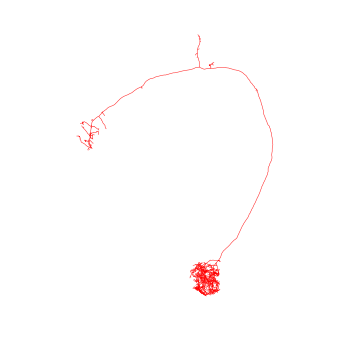# Plotting

Explore how to plot neurons in 2d using and in 3d

# Plotting

navis lets you plot neurons in 2d using matplotlib (nice for figures), and in 3d using either plotly when in a notebook environment like Deepnote or using a vispy-based 3D viewer when using a Python terminal. The vispy solution won’t work in Deepnote so we will focus on matplotlib’s 2d and plotly for 3d.

import navis

# This is relevant because Deepnote does not (yet) support fancy progress bars
navis.set_pbars(jupyter=False)

# Load one of the example neurons shipped with navis
n = navis.example_neurons(1, kind='skeleton')

WARNING: Could not load OpenGL library.

# Make a 2d plot
fig, ax = navis.plot2d(n)

# Note that this is equivalent to
# fig, ax = n.plot2d()If you have seen an olfactory projection neuron before, you might have noticed that this neuron is upside-down. That’s because hemibrain neurons have an odd orienation in that the anterior-posterior axis not the z- but the y-axis (they have been image from above).

For us that just means we have to turn the camera ourselves if we want a frontal view:

# Make a 2d plot
fig, ax = navis.plot2d(n)

# Change camera (azimuth + elevation)
ax.azim, ax.elev = -90, -90Let’s do the same in 3d:

# Get a list of neurons
nl = navis.example_neurons(5)

# Plot
navis.plot3d(nl, width=1000)


• left click and drag to rotate (select “Orbital rotation” above the legend to make your life easier)
• mousewheel to zoom
• middle-mouse + drag to translate
• click legend items (single or double) to hide/unhide

Above plots are very basic examples but there are a ton of ways to tweak things to your liking. For a full list of parameters check out the docs for plot2d and plot3d.

Let’s for example change the colors. In general, colors can be:

• a string - e.g. "red" or just "r"
• an rgb/rgba tuple - e.g. (1, 0, 0) for red
# Plot all neurons in red
fig, ax = navis.plot2d(n, color='r')
ax.azim, ax.elev = -90, -90# Plot all neurons in red (color as tuple)
fig, ax = navis.plot2d(n, color=(1, 0, 0, 1))
ax.azim, ax.elev = -90, -90When plotting multiple neurons you can either use:

• a single color ("r" or (1, 0, 0)) -> assigned to all neurons
• a list of colors (['r', 'yellow', (0, 0, 1)]) with a color for each neuron
• a dictionary mapping neuron IDs to colors ({1734350788: 'r', 1734350908: (1, 0, 1)})
• the name of a matplotlib or seaborn color palette
# Plot with a specific color palette
navis.plot3d(nl, color='jet')